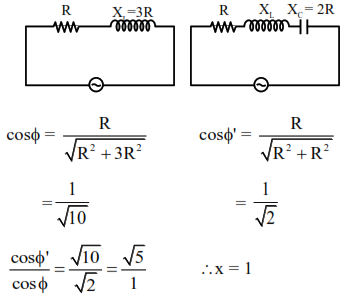# An ac circuit has an inductor`
Question:

An ac circuit has an inductor and a resistor of resistance $\mathrm{R}$ in series, such that $\mathrm{X}_{\mathrm{L}}=3 \mathrm{R}$. Now, a capacitor is added in series such that $X_{C}=2 R$. The ratio of new power factor with the old power factor of the circuit is $\sqrt{5}: \mathrm{x}$. The value of $\mathrm{x}$ is

Solution: